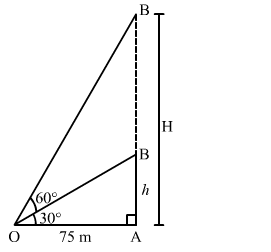# The angle of elevation of the top of an unfinished tower at a distance of 75 m form its base is 30°.`
Question:

The angle of elevation of the top of an unfinished  tower at a distance of 75 m form its base is 30°. How much higher must the tower be raised so that the angle of elevation of its top at the same point may be 60°?

Solution:

Let $A B$ be the unfinished tower, $A C$ be the raised tower and $\mathrm{O}$ be the point of observation.

We have:

$O A=75 \mathrm{~m} \cdot \angle A O B=30^{\circ}$ and $\angle A O C=60^{\circ}$

Let $A C=H \mathrm{~m}$ such that $B C=(H-h) \mathrm{m}$.In ∆AOB, we have:

$\frac{A B}{O A}=\tan 30^{\circ}=\frac{1}{\sqrt{3}}$

$\Rightarrow \frac{h}{75}=\frac{1}{\sqrt{3}}$

$\Rightarrow h=\frac{75}{\sqrt{3}} \mathrm{~m}=\frac{75 \times \sqrt{3}}{\sqrt{3} \times \sqrt{3}}=25 \sqrt{3} \mathrm{~m}$

In $\triangle A O C$, we have:

$\frac{A C}{O A}=\tan 60^{\circ}=\sqrt{3}$

$\Rightarrow \frac{H}{75}=\sqrt{3}$

$\Rightarrow H=75 \sqrt{3} \mathrm{~m}$

$\therefore$ Required height $=(H-h)=(75 \sqrt{3}-25 \sqrt{3})=50 \sqrt{3} \mathrm{~m}=86.6 \mathrm{~m}$